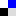mathematical expressions
rating: 0

\ˌmath-ˈma-ti-kəl, ˌma-thə-\ \ik-ˈspre-shən\\

Etymology: mathematical (Middle English mathematicalle, from Latin mathematicus, from Greek mathēmatikos, from mathēmat-, mathēma learning, mathematics, from manthanein to learn; probably akin to Gothic mundon to pay attention) + expressions (Middle English, from Anglo-French expres, from Latin expressus, past participle of exprimere to press out, express, from ex- + premere to press)
Function: noun

1: near-reality mathematical abstractions which are used so as to understand the expansion and the development of the cities, through cellular automata models and agent-based simulations.  

Bibliography
1. “Cities and complexity: understanding cities with cellular automata, agent-based models, and fractals.” Michael Batty (2005)
Tag this site indel.icio.us.